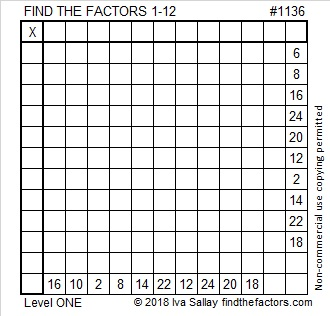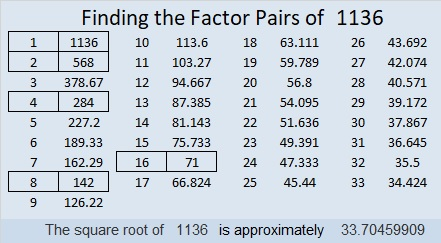# 1136 and Level 1

This puzzle has 20 clues to help you to know where to write the factors 1 to 12 in both the first column and the top row. After you find all the factors you can make the puzzle be a special type of multiplication table.Print the puzzles or type the solution in this excel file: 12 factors 1134-1147

Here are a few facts about the number 1136:

• 1136 is a composite number.
• Prime factorization: 1136 = 2 × 2 × 2 × 2 × 71, which can be written 1136 = 2⁴ × 71
• The exponents in the prime factorization are 4 and 1. Adding one to each and multiplying we get (4 + 1)(1 + 1) = 5 × 2 = 10. Therefore 1136 has exactly 10 factors.
• Factors of 1136: 1, 2, 4, 8, 16, 71, 142, 284, 568, 1136
• Factor pairs: 1136 = 1 × 1136, 2 × 568, 4 × 284, 8 × 142, or 16 × 71
• Taking the factor pair with the largest square number factor, we get √1136 = (√16)(√71) = 4√71 ≈ 33.70461136 is palindrome 2C2 in BASE 21 (C is 12 base 10)
because 2(21²) + 12(21) + 2(1) = 1136

This site uses Akismet to reduce spam. Learn how your comment data is processed.